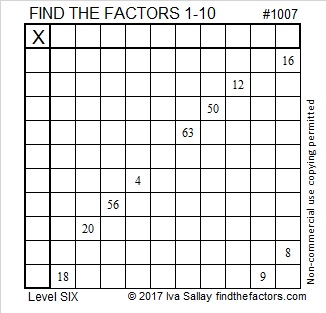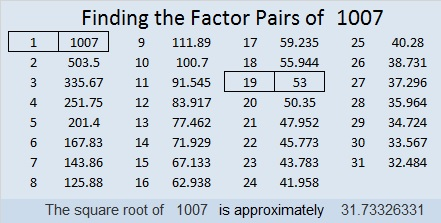# 1007 and Level 6

Some of the clues in this puzzle pair up in the same column or the same row and try to trick you into picking the wrong common factor. Nevertheless, the 10 clues in the puzzle work together to give you the most logical place to start the puzzle. It may be a little difficult to see the logic for this one but stick with it. You’ll figure it out.Print the puzzles or type the solution in this excel file: 10-factors-1002-1011

Here’s a little bit about the number 1007:

1007 is the hypotenuse of a Pythagorean triple:
532-855-1007lwhich is 19 times (28-45-53)

1007 is palindrome 33233 in BASE 4
because 3(4⁴) + 3(4³) + 2(4²) + 3(4¹) + 3(4⁰) = 1007

• 1007 is a composite number.
• Prime factorization: 1007 = 19 × 53
• The exponents in the prime factorization are 1 and 1. Adding one to each and multiplying we get (1 + 1)(1 + 1) = 2 × 2 = 4. Therefore 1007 has exactly 4 factors.
• Factors of 1007: 1, 19, 53, 1007
• Factor pairs: 1007 = 1 × 1007 or 19 × 53
• 1007 has no square factors that allow its square root to be simplified. √1007 ≈ 31.73326This site uses Akismet to reduce spam. Learn how your comment data is processed.